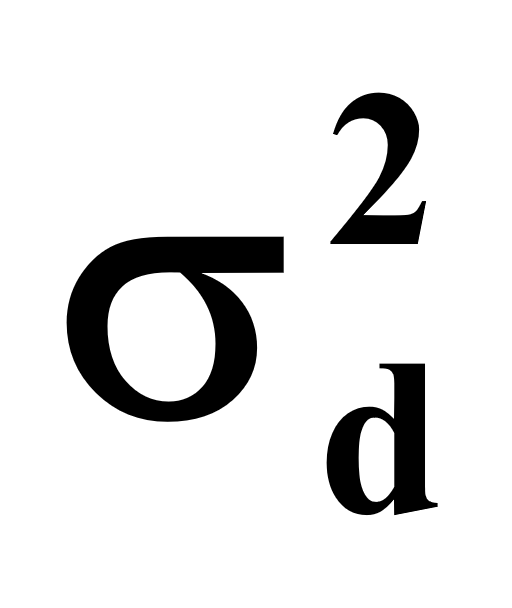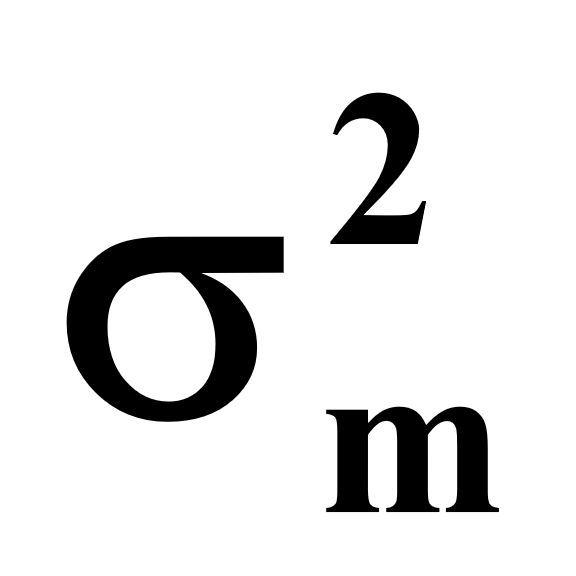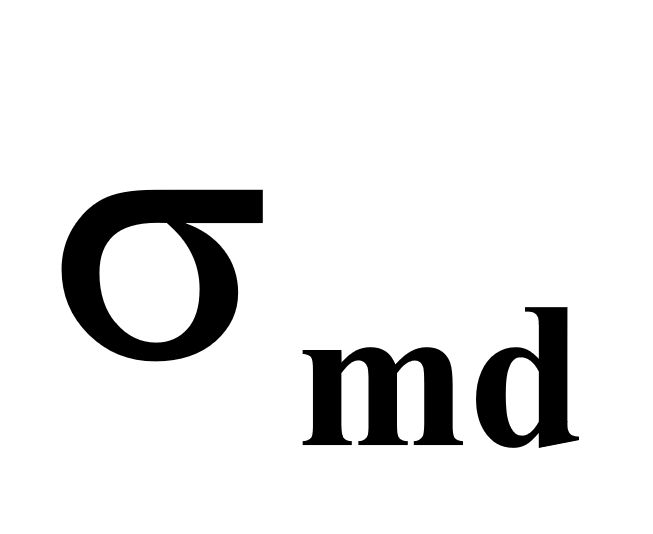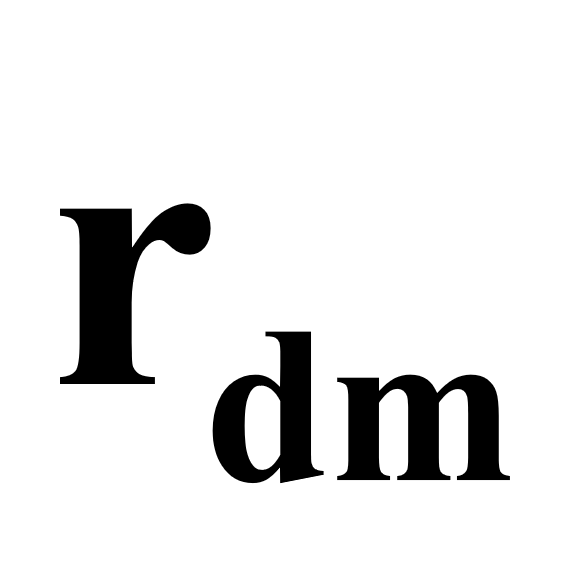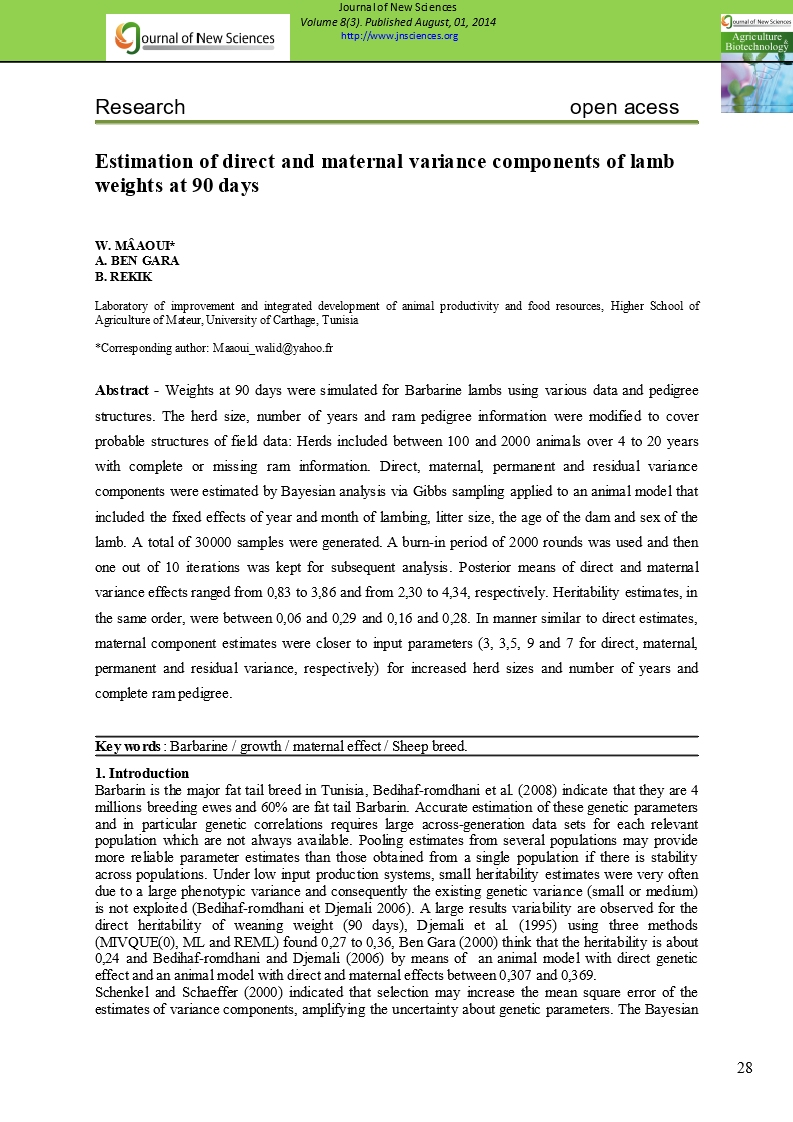# Estimation of direct and maternal variance components of lamb weights at 90 days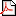Volume 8, Article 3 [Volume 8, Article 3] 541 kB

W. Mâaoui*

A. Ben Gara

B. Rekik

Laboratory of improvement and integrated development of animal productivity and food resources, Higher School of Agriculture of Mateur, University of Carthage, Tunisia

Abstract - Weights at 90 days were simulated for Barbarine lambs using various data and pedigree structures. The herd size, number of years and ram pedigree information were modified to cover probable structures of field data: Herds included between 100 and 2000 animals over 4 to 20 years with complete or missing ram information. Direct, maternal, permanent and residual variance components were estimated by Bayesian analysis via Gibbs sampling applied to an animal model that included the fixed effects of year and month of lambing, litter size, the age of the dam and sex of the lamb. A total of 30000 samples were generated. A burn-in period of 2000 rounds was used and then one out of 10 iterations was kept for subsequent analysis. Posterior means of direct and maternal variance effects ranged from 0,83 to 3,86 and from 2,30 to 4,34, respectively. Heritability estimates, in the same order, were between 0,06 and 0,29 and 0,16 and 0,28. In manner similar to direct estimates, maternal component estimates were closer to input parameters (3, 3,5, 9 and 7 for direct, maternal, permanent and residual variance, respectively) for increased herd sizes and number of years and complete ram pedigree.

Key words: Barbarine / growth / maternal effect / Sheep breed.

1. Introduction

Barbarin is the major fat tail breed in Tunisia, Bedihaf-romdhani et al. (2008) indicate that they are 4 millions breeding ewes and 60% are fat tail Barbarin. Accurate estimation of these genetic parameters and in particular genetic correlations requires large across-generation data sets for each relevant population which are not always available. Pooling estimates from several populations may provide more reliable parameter estimates than those obtained from a single population if there is stability across populations. Under low input production systems, small heritability estimates were very often due to a large phenotypic variance and consequently the existing genetic variance (small or medium) is not exploited (Bedihaf-romdhani et Djemali 2006). A large results variability are observed for the direct heritability of weaning weight (90 days), Djemali et al. (1995) using three methods (MIVQUE(0), ML and REML) found 0,27 to 0,36, Ben Gara (2000) think that the heritability is about 0,24 and Bedihaf-romdhani and Djemali (2006) by means of an animal model with direct genetic effect and an animal model with direct and maternal effects between 0,307 and 0,369.

Schenkel and Schaeffer (2000) indicated that selection may increase the mean square error of the estimates of variance components, amplifying the uncertainty about genetic parameters. The Bayesian methods can fully take into account the uncertainty about dispersion parameters by considering the marginal posterior density of those parameters (Gianola and Fernando1986; Wang et al.1993 and Sorensen et al., 1994)

Gibbs sampling is a Monte Carlo numerical integration method that allows inferences to be made about joint or marginal distributions, even if appropriate densities cannot be explicitly formed (Geman and Geman 1984). The great appeal of the Bayesian analyses via Gibbs sampling is that it yields Monte Carlo estimates of the full marginal posterior distribution of all parameters of interest, for instance breeding values, from which the probabilities that the parameter lies between specified values can be computed (Van Tassel et al.1994; Sorensen 1996).

The cycle of generating each parameter is repeated. Eventually, the Gibbs sampler converges to the posterior distribution, and the values drawn after that convergence are considered random samples from the posterior distribution. The number of rounds discarded before the values are considered samples from the posterior distribution is usually called the burn-in period (Van Tassell and Van Vleck 1996).

Bayesian analyses via Gibbs sampling are becoming more and more feasible as computer power increases and as better algorithms are developed. The applicability of Bayesian methods for genetic evaluation is already possible routinely for moderately sized problems (Schenkel et al. 2002).

The objective of this study was to determinate estimated variance components of lamb growth at 90 days with a simulated data (different scenarios) and using a Markov Chain Monte Carlo Bayesian method.

2. Materials and methods

2.1. Data Simulation

Simulation of a Barbarin Herd:

- Fertility: 0,9

- Prolificacy: 1,3

- Mortality: 0,0

- Initial herd size: N

- Sex ratio: 0,05

Variance components:

- Direct genetic additive variance: 3 Kg2

- Maternal genetic additive variance: 3,5 Kg2

- Direct-maternal covariance: -0,5 Kg2

- Permanent environmental effect variance: 1 Kg2

- Residual variance: 7 Kg2

- h2d: 0,207

- h2m: 0,241

Animal genetic value of the base herd: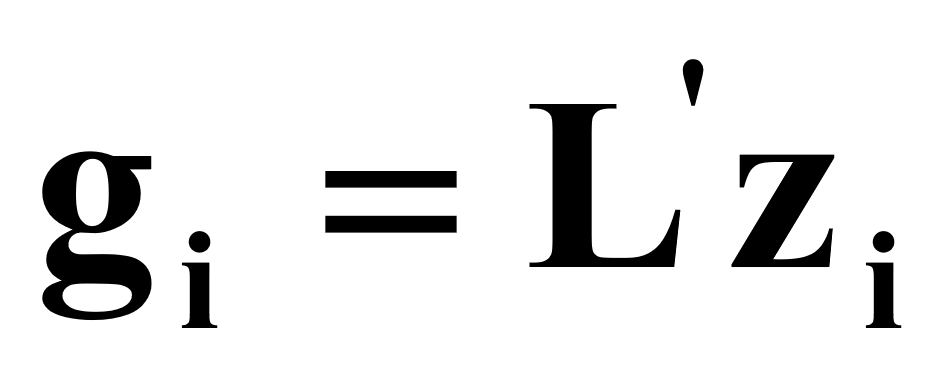i= 1,……,N

With L’ such as G0=L’L and~N(02, 12)

The permanent maternal effect :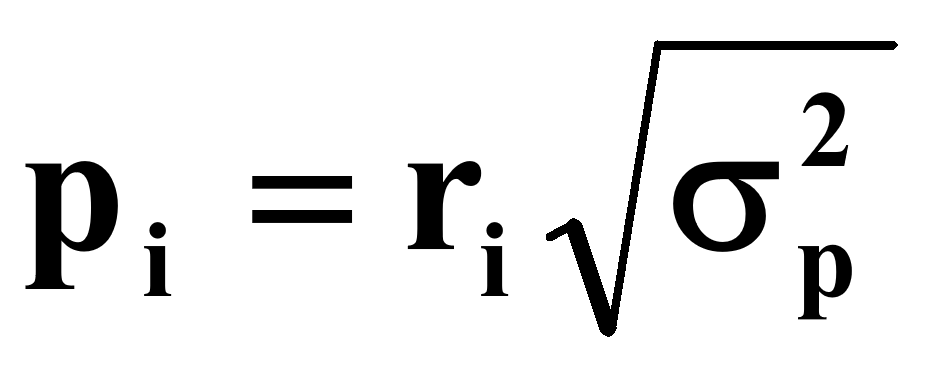With r~N(0,1)

Lambing year’s effect is determinate like this:

an~N(0,1) and the effect of month, sex and type of birth and age of the ewe are taken from a Tunisian Barbarin semi-arid results (Ben Gara, no published references).

Descendant generation’s simulation:

The descendants come from hazard mating of the reproductive animals kept every year with a phenotypic selection. The yearly reform rate is about 17%.

The breeding values of a descendant are calculated like this:

Then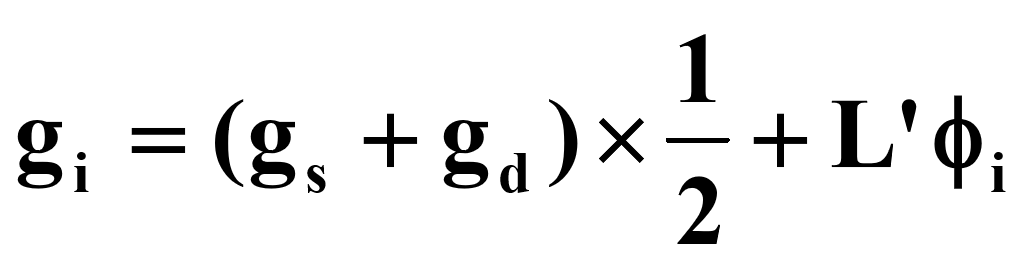with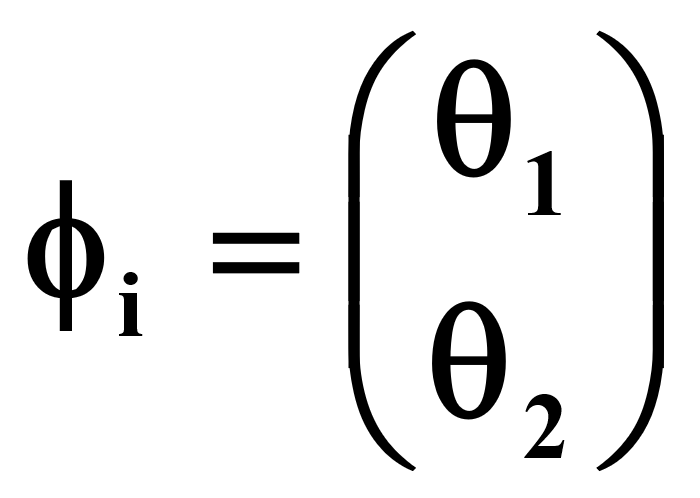et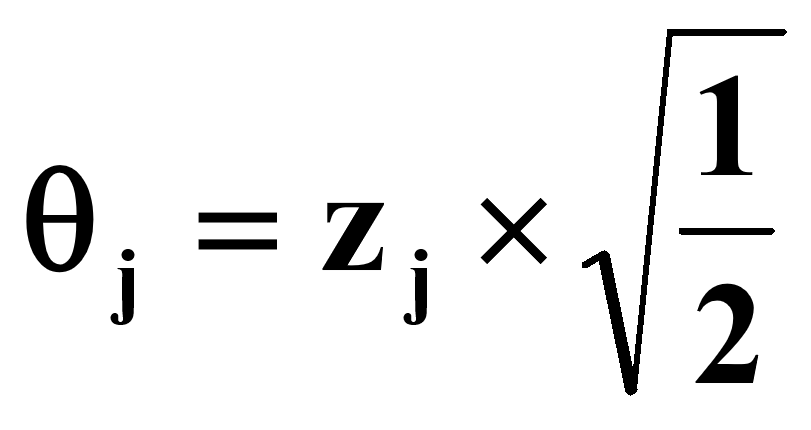where zj~N(0,1)

s and d are respectively the sire and the dam of the animal i.

The phenotypic value of the animal i is calculated in this way:

Phi=gi(1) + gd(2) + pd + ei + Σfi

With gi(1) Direct genetic additive effect of the animal i

gd(2) Maternal genetic additive variance effect of the dam d

pd permanent environmental effect of the dam d

ei residual value of the animal i

and Σfi The whole of fixed effects affecting the performance of the animal i (year, month, sex and type of birth , age of the ewe).

With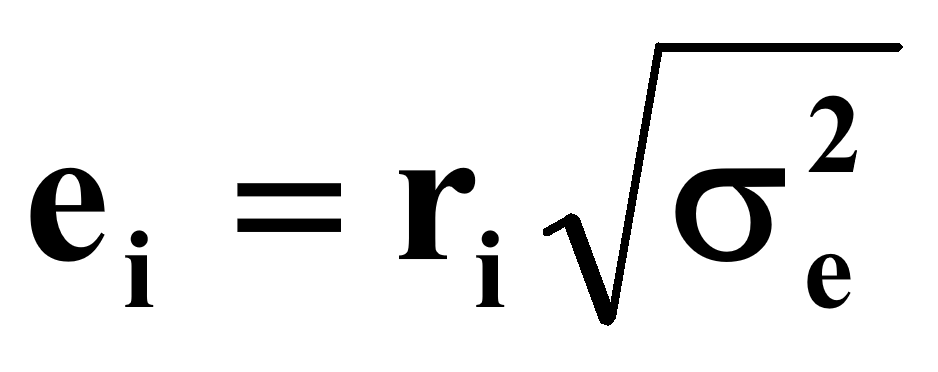and ri~N(0,1)

The table below gives us an idea about the number of lambs simulated, the base herd range between 100 and 2000, the number of years is between 4 and 20. Example for a base herd of 100 ewes and with 4 years of reproduction we obtain 495 lambs.

 Table 1. Description of the data simulated Base herd Number of years 4 6 8 10 12 20 100 250 300 500 1000 1200 1500 2000 495 1299 1518 2478 5025 6097 7446 10175 700 1859 2163 3534 7147 8684 10606 14645 914 2,398 2,819 4,597 9372 11414 13887 19106 1131 2969 3487 5693 11645 14120 17243 23698 1356 3509 4167 6826 13874 16833 20613 28280 2260 5714 6883 11364 22944 27716 34192 46478

2.2. Analyses

The linear mixed model used in this analysis was:

y= Xb + Wp + Zmm + Zaa +e (1)

With W = Zm

m : Vector of maternal genetic additive effect q

a : Vector of direct genetic additive effect q

p : Vector permanent environnemental effect p

N : Number of observation

We define :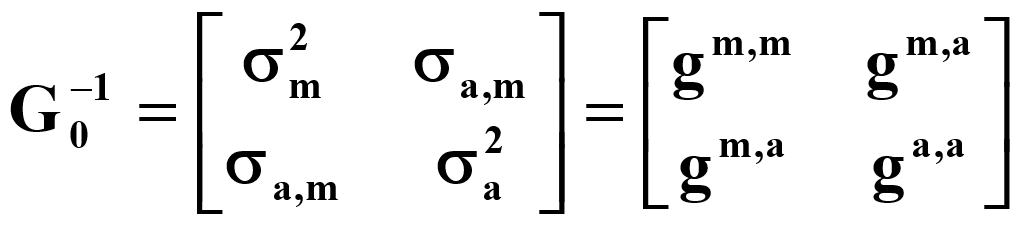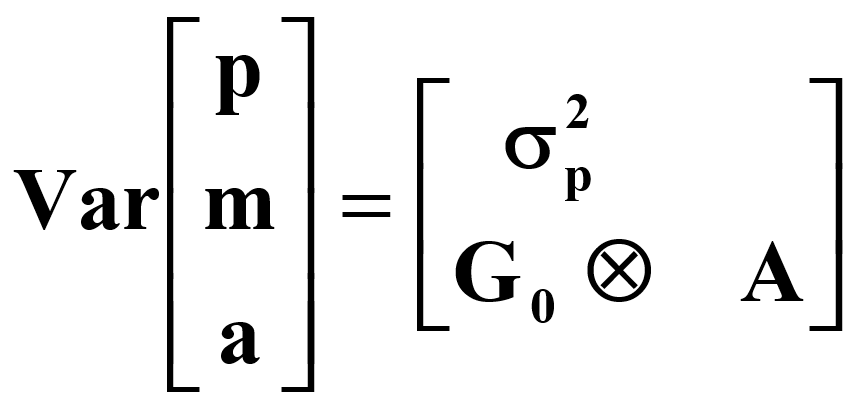withproduit de Kronecker

y~N(Xb + Wp + Zmm + Zaa, I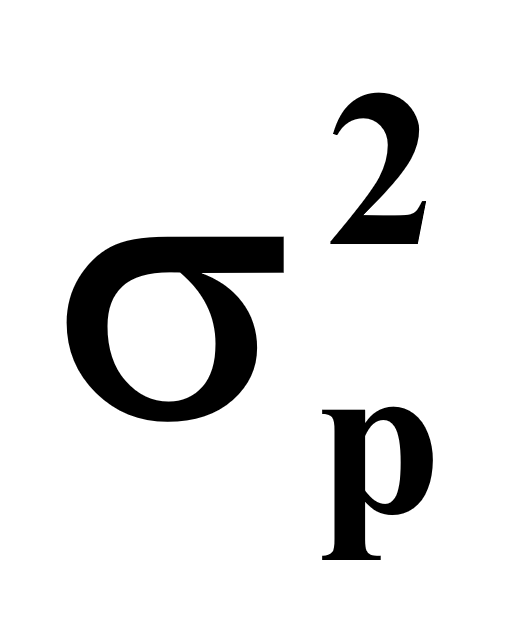)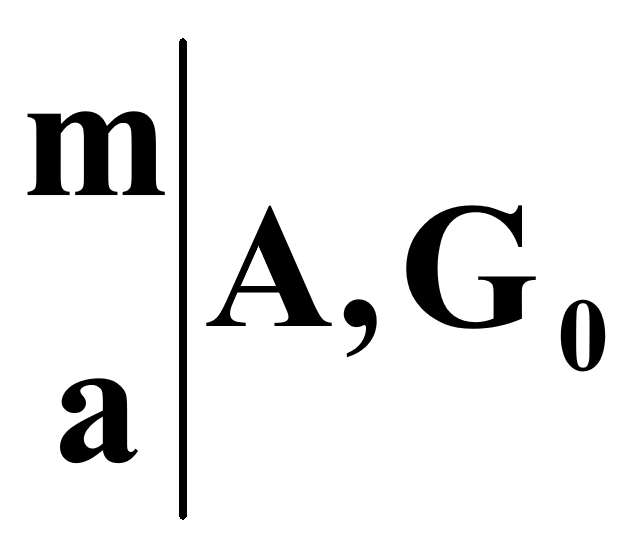~N(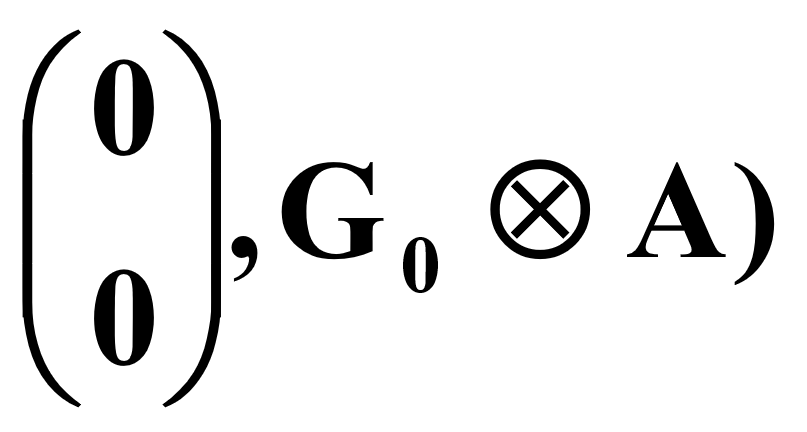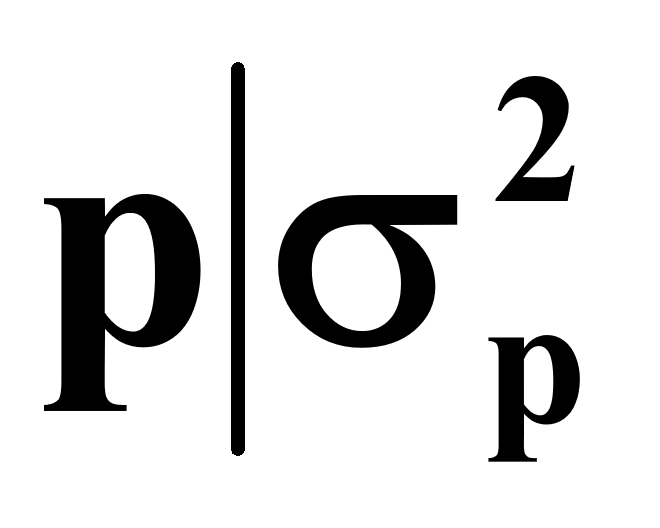~N(0,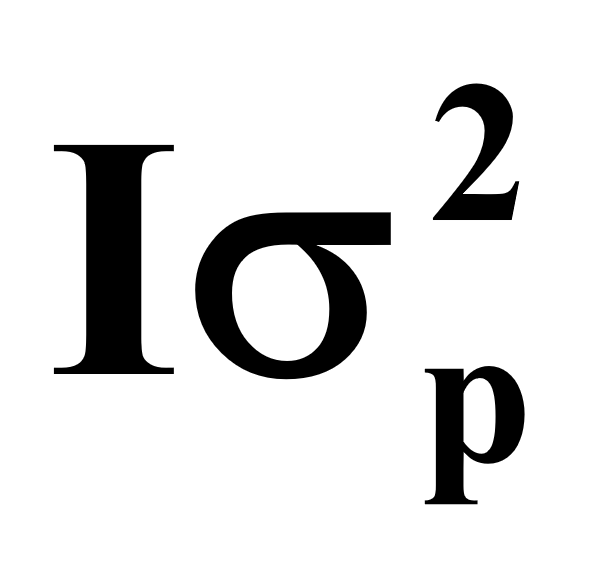)

g’=(m’a’) with 2 q order.A priori distribution definition

p(b)~ a constant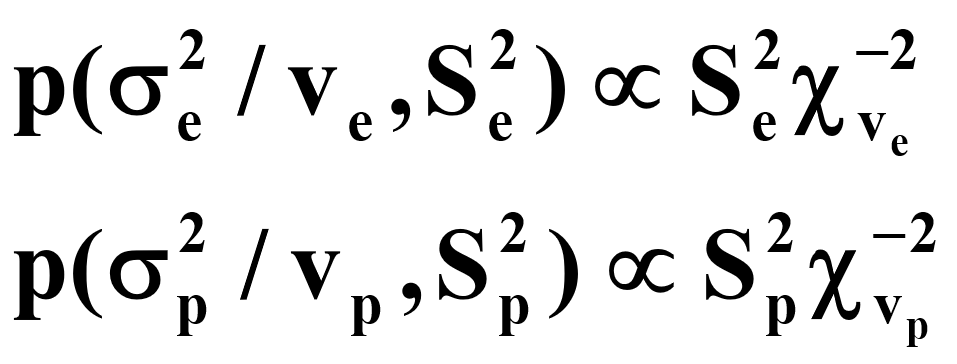p(G0/V,v)IW2(V,v)

with v=-3 , V=0 in this way p(G0/V,v) is a uniform distribution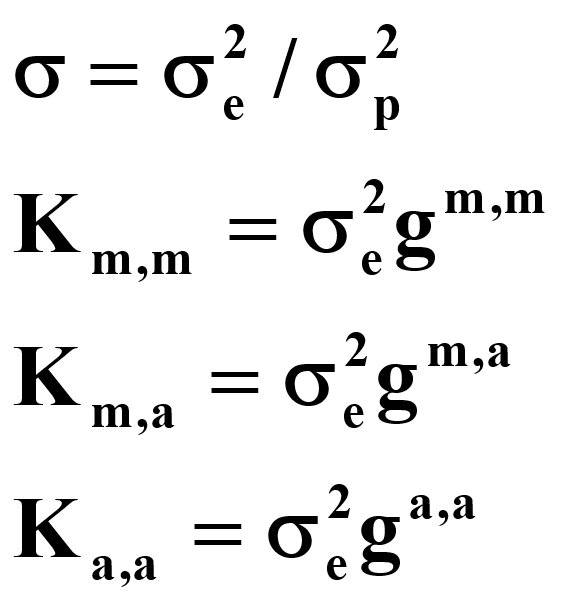Xb + Zmp + Zmm + Zaa = Wθ

Then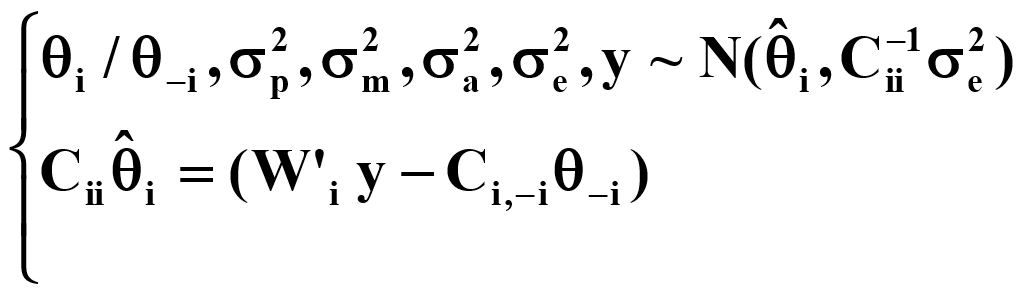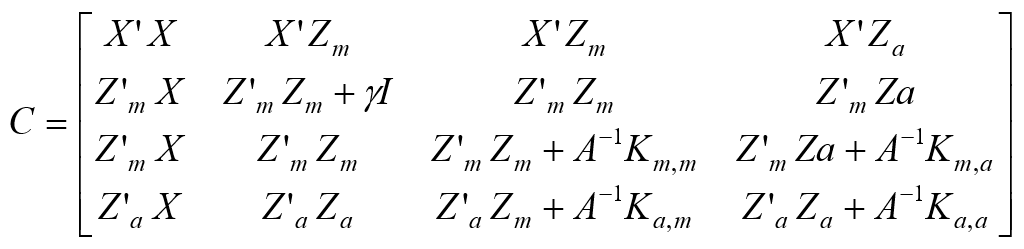I

With :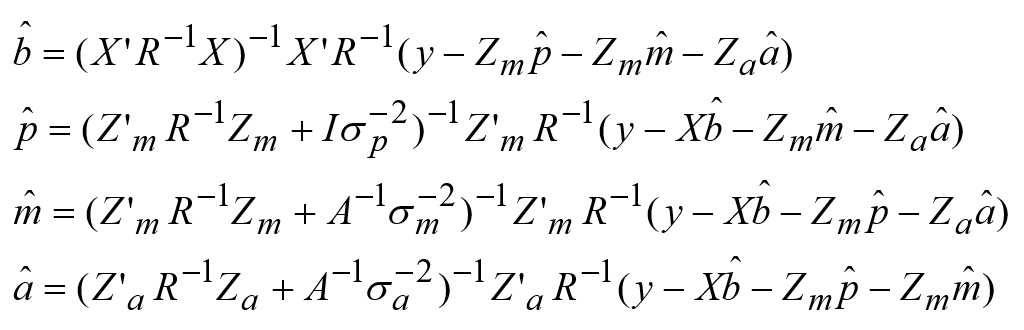With

b~N(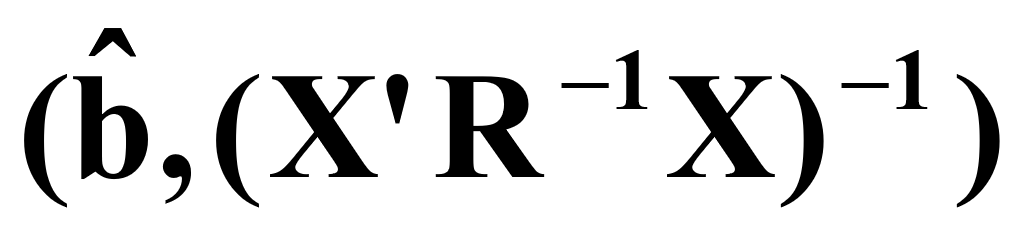p~N(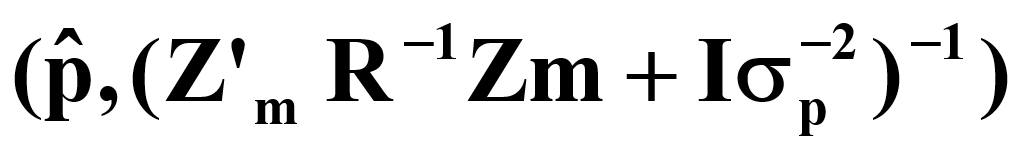m~N(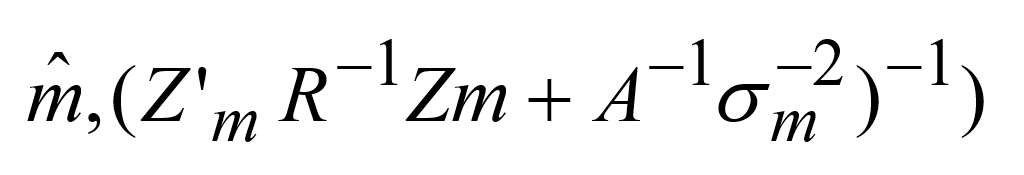a~N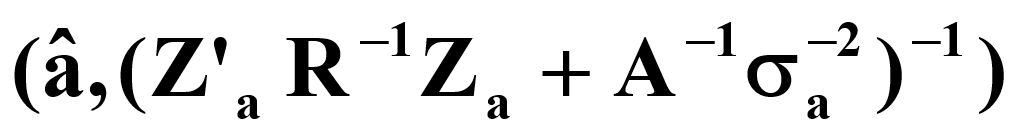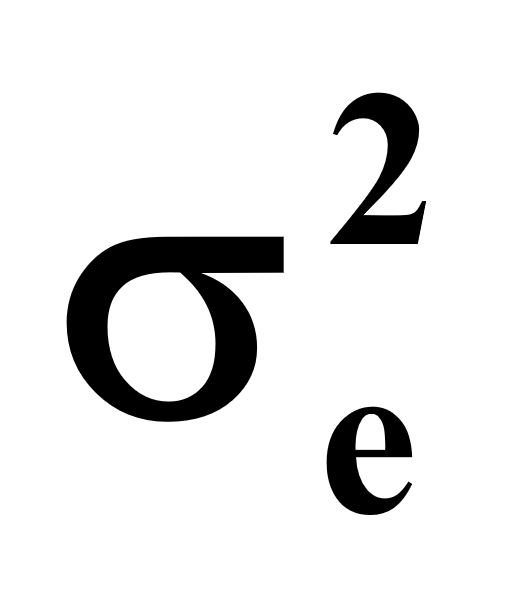~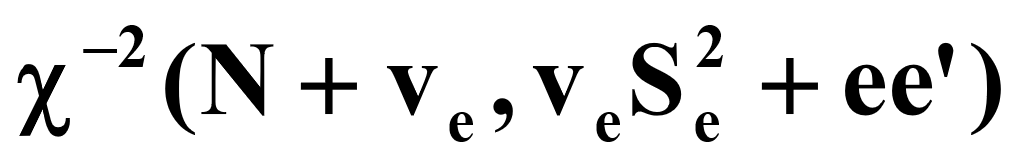~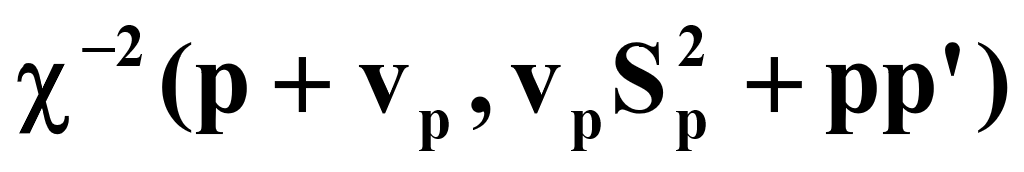G0~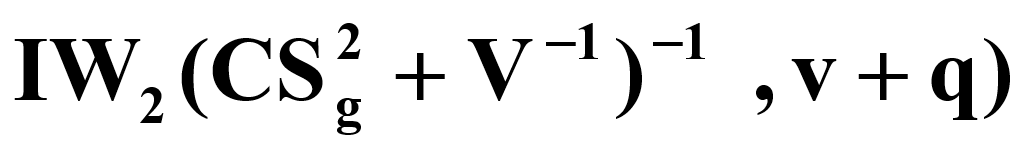And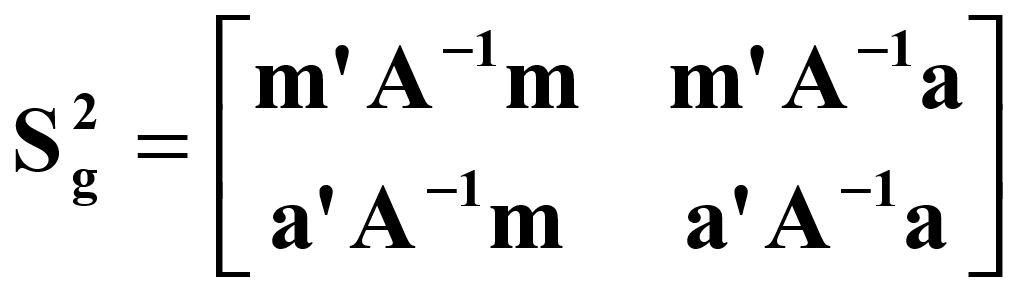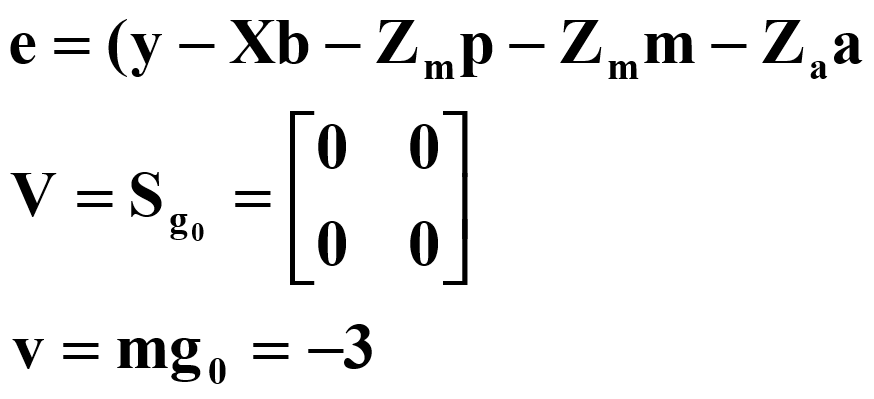A Wishart distribution simulation

Wp(S,n)

We proceed with a Chodesky factorisation :

S=L’L

We build an inferior triangle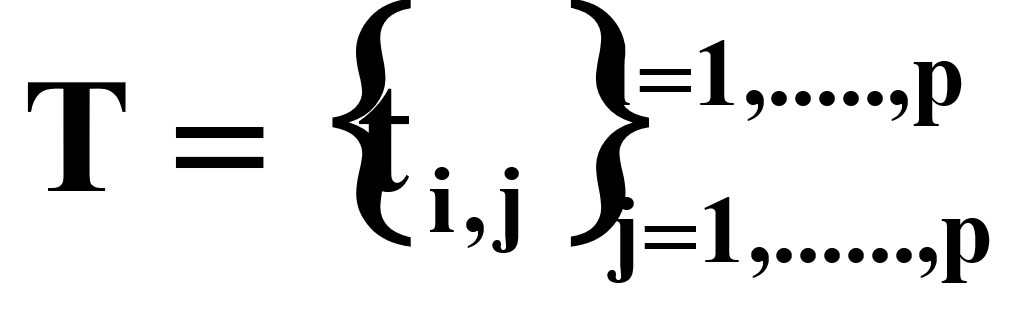With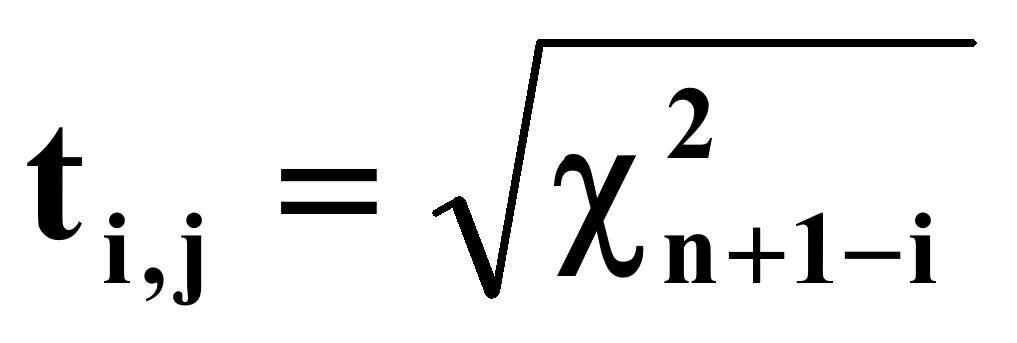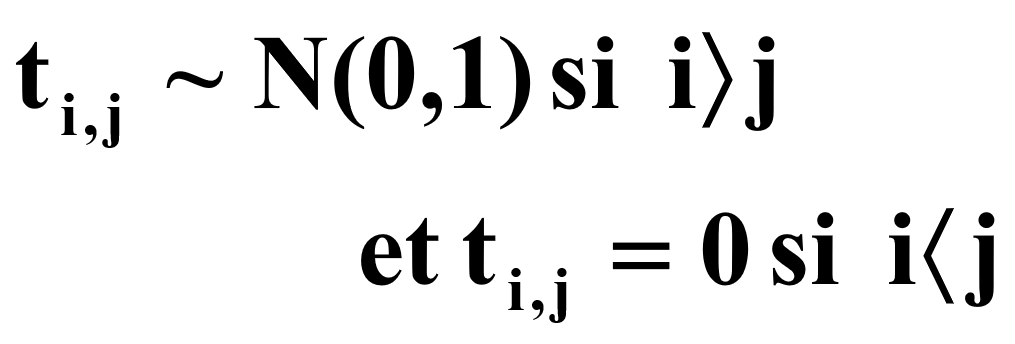Then Q=L’TT’L~Wp(S,n)

And Q-1~I Wp(S,n)

The hyper parameter V(prior) is determinated in this way :

E(G0/V,v)=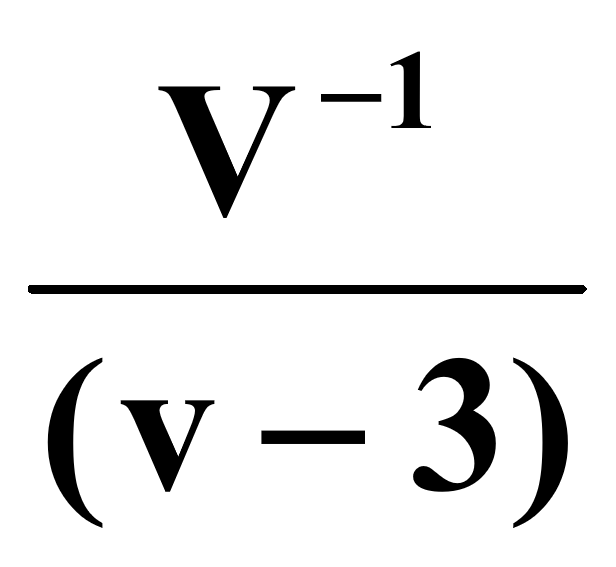We choose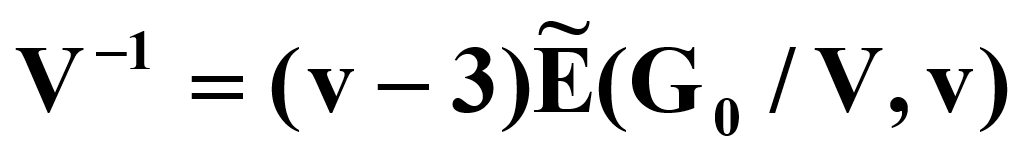And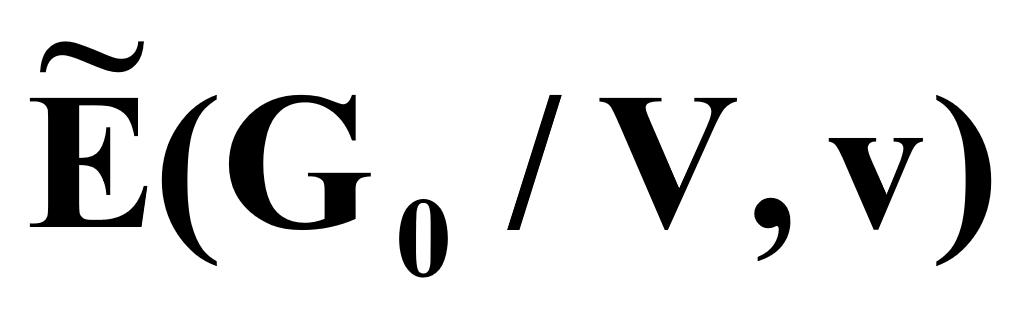establish the a priori information base.

3. Results and discussions

3.1. Variance component estimates

Marginal distributions were used with herd’s size 100 and 500 to determine: direct, maternal, permanent environment and residual variances. Estimated posterior and standard deviation of all of these criteria are presented in Table 2. Posterior means for direct variance effect ranged from 0,83 to 3,83. This estimate is similar with Mandal et al. (2006) results who reported 3,23 for pre-weaning weight (75 days) with a Muzaffarnagari sheep. A similar result (3,99) was founded by Mokhtari et al. (2008) for weight at 6 months of Kermani sheep. A lower direct genetic variance for the weight at 90 days (0.57) was estimated by an animal model with direct and maternal effects with barbarin lambs (Bedhiaf-Romdhani and Djemali 2006).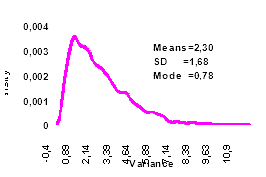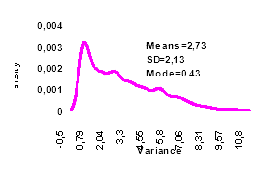Figure 1. Posterior density function of direct variance (T100-G4) Complete ram information Figure 2. Posterior density function of direct variance (T100-SG-G4) sire ram missing information

Marginal distributions of direct variance for a herd size of 100 ewes and 4 years with complete ram information (T100-G4) and a sire ram missing information (T100-SG-G4) are presented in Figures. 1-2, respectively. Direct variance ranged from -0,4 to 11,6 for the first design and from -0,5 to 10,9 for the second. With complete ram information the mode is about 0,78 and 0,43 with a sire ram missing information.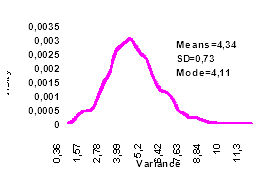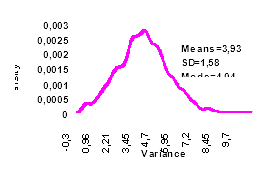Figure 3. Posterior density function of maternal variance (T100-G4) Complete ram information Figure 4. Posterior density function of direct variance (T100-SG-G4) sire ram missing information

 Table 2. Estimated posterior and standard deviation of direct, maternal, permanent environment and residual variances Design